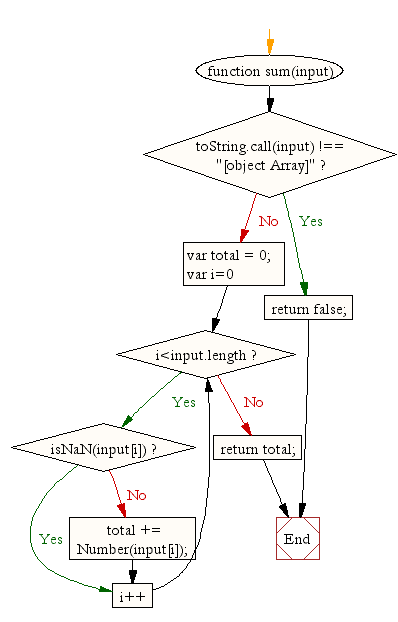# JavaScript: Calculate the sum of values in an array

## JavaScript Math: Exercise-17 with Solution

Write a JavaScript function to calculate the sum of values in an array.

Test Data :
console.log(sum([1,2,3]));
console.log(sum([100,-200,3]));
console.log(sum([1,2,'a',3]));
Output :
6
-97
6

Pictorial Presentation:Sample Solution:-

HTML Code:

``````<!DOCTYPE html>
<html>
<meta charset="utf-8">
<title>Sum of values in an array</title>
<body>
</body>
</html>
```
```

JavaScript Code:

``````function sum(input){

if (toString.call(input) !== "[object Array]")
return false;

var total =  0;
for(var i=0;i<input.length;i++)
{
if(isNaN(input[i])){
continue;
}
total += Number(input[i]);
}
}
console.log(sum([1,2,3]));
console.log(sum([100,-200,3]));
console.log(sum([1,2,'a',3]));
```
```

Sample Output:

```6
-97
6
```

Flowchart:Live Demo:

See the Pen javascript-math-exercise-17 by w3resource (@w3resource) on CodePen.

Improve this sample solution and post your code through Disqus

What is the difficulty level of this exercise?

Test your Programming skills with w3resource's quiz.

﻿